# More on Models and Numerical Procedures Chapter 26

• Slides: 46
Download presentation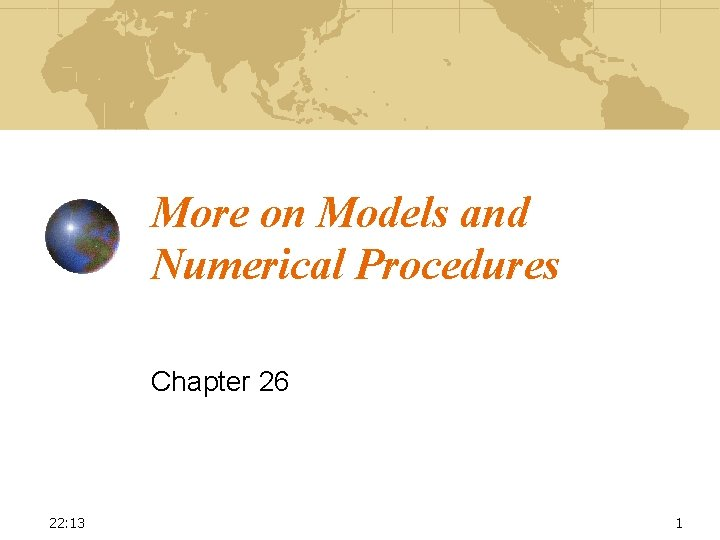More on Models and Numerical Procedures Chapter 26 22: 13 1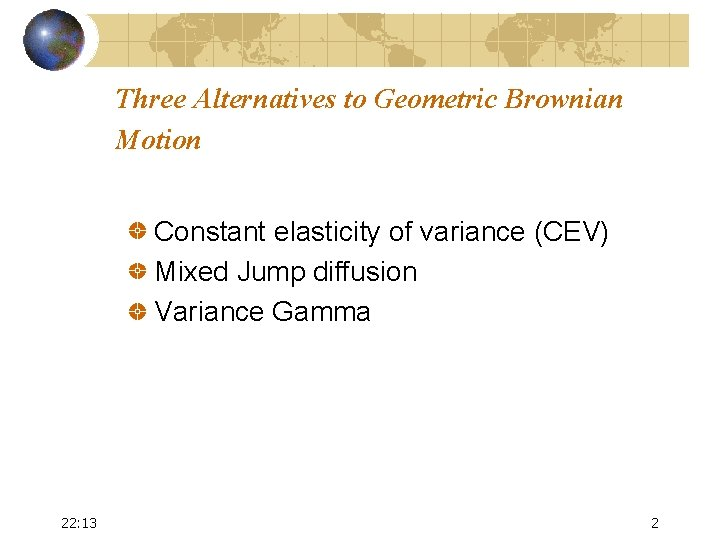Three Alternatives to Geometric Brownian Motion Constant elasticity of variance (CEV) Mixed Jump diffusion Variance Gamma 22: 13 2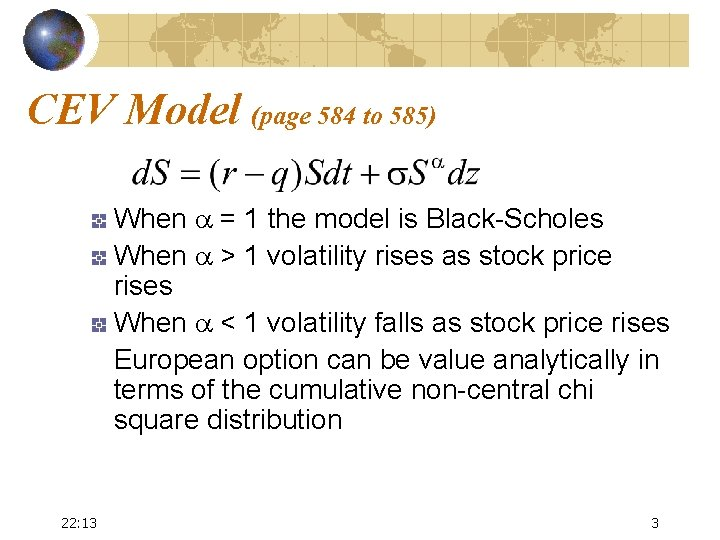CEV Model (page 584 to 585) When a = 1 the model is Black-Scholes When a > 1 volatility rises as stock price rises When a < 1 volatility falls as stock price rises European option can be value analytically in terms of the cumulative non-central chi square distribution 22: 13 3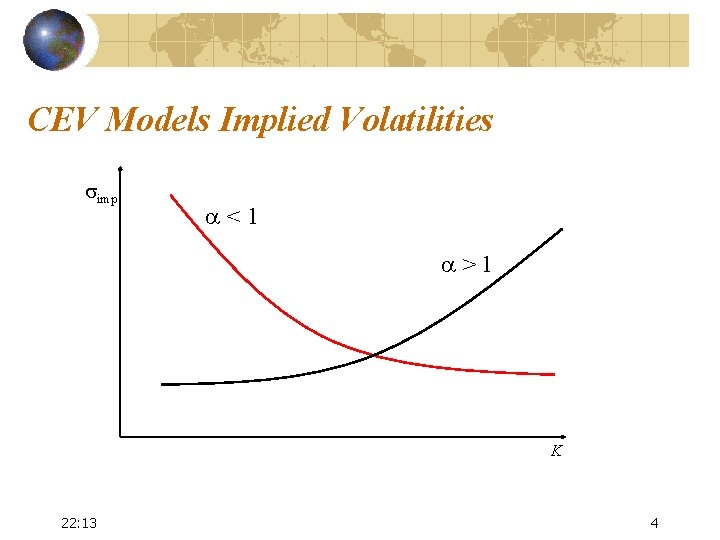CEV Models Implied Volatilities simp a<1 a>1 K 22: 13 4Mixed Jump Diffusion Model (page 585 to 586) Merton produced a pricing formula when the asset price follows a diffusion process overlaid with random jumps dp is the random jump k is the expected size of the jump l dt is the probability that a jump occurs in the next interval of length dt 22: 13 5Jumps and the Smile Jumps have a big effect on the implied volatility of short term options They have a much smaller effect on the implied volatility of long term options 22: 13 6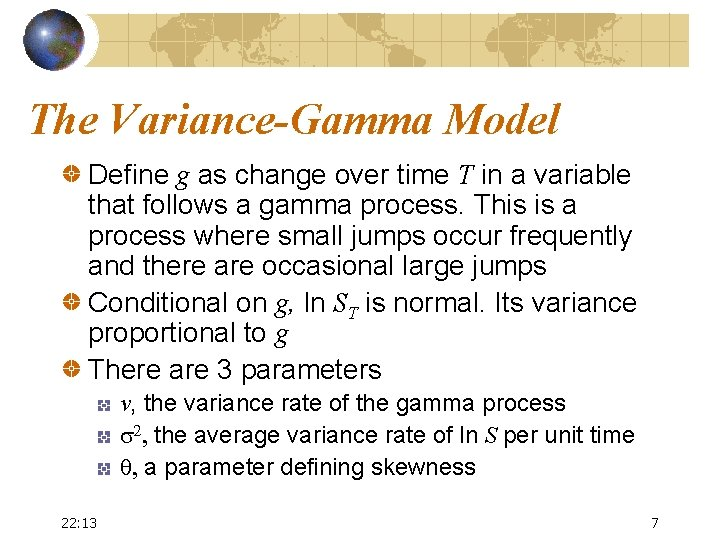The Variance-Gamma Model Define g as change over time T in a variable that follows a gamma process. This is a process where small jumps occur frequently and there are occasional large jumps Conditional on g, ln ST is normal. Its variance proportional to g There are 3 parameters v, the variance rate of the gamma process s 2, the average variance rate of ln S per unit time q, a parameter defining skewness 22: 13 7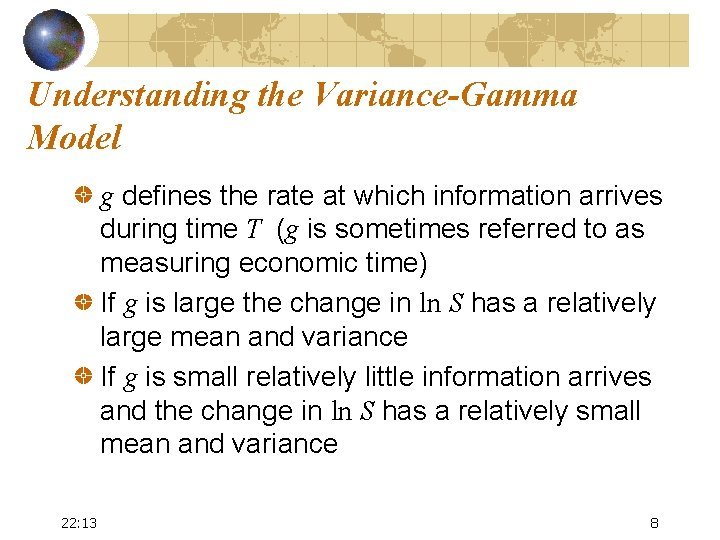Understanding the Variance-Gamma Model g defines the rate at which information arrives during time T (g is sometimes referred to as measuring economic time) If g is large the change in ln S has a relatively large mean and variance If g is small relatively little information arrives and the change in ln S has a relatively small mean and variance 22: 13 8Time Varying Volatility Suppose the volatility is s 1 for the first year and s 2 for the second and third Total accumulated variance at the end of three years is s 12 + 2 s 22 The 3 -year average volatility is 22: 13 9Stochastic Volatility Models (equations 26. 2 and 26. 3, page 589) When V and S are uncorrelated a European option price is the Black-Scholes price integrated over the distribution of the average variance 22: 13 10Stochastic Volatility Models continued When V and S are negatively correlated we obtain a downward sloping volatility skew similar to that observed in the market for equities When V and S are positively correlated the skew is upward sloping. (This pattern is sometimes observed for commodities) 22: 13 11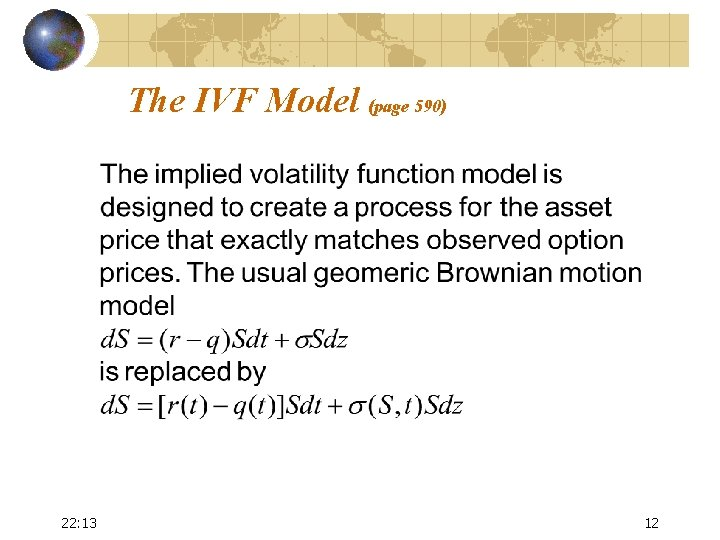The IVF Model (page 590) 22: 13 12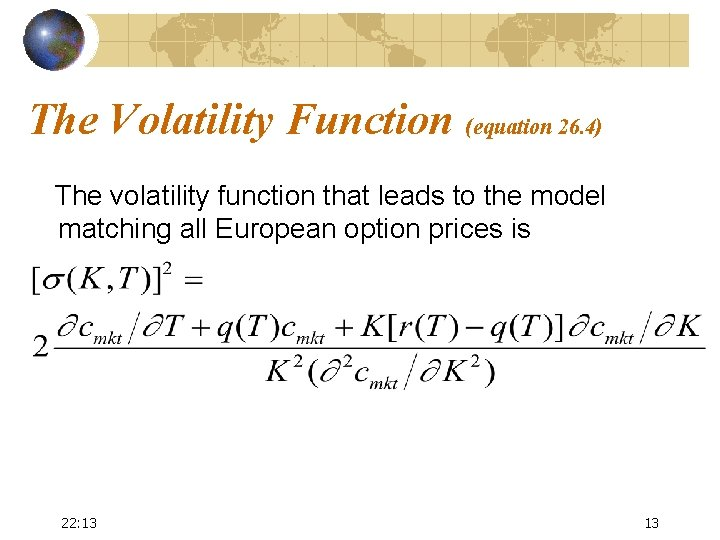The Volatility Function (equation 26. 4) The volatility function that leads to the model matching all European option prices is 22: 13 13Strengths and Weaknesses of the IVF Model The model matches the probability distribution of asset prices assumed by the market at each future time The models does not necessarily get the joint probability distribution of asset prices at two or more times correct 22: 13 14Convertible Bonds Often valued with a tree where during a time interval Dt there is a probability pu of an up movement A probability pd of a down movement A probability 1 -exp(-lt) that there will be a default (l is the hazard rate) In the event of a default the stock price falls to zero and there is a recovery on the bond 22: 13 15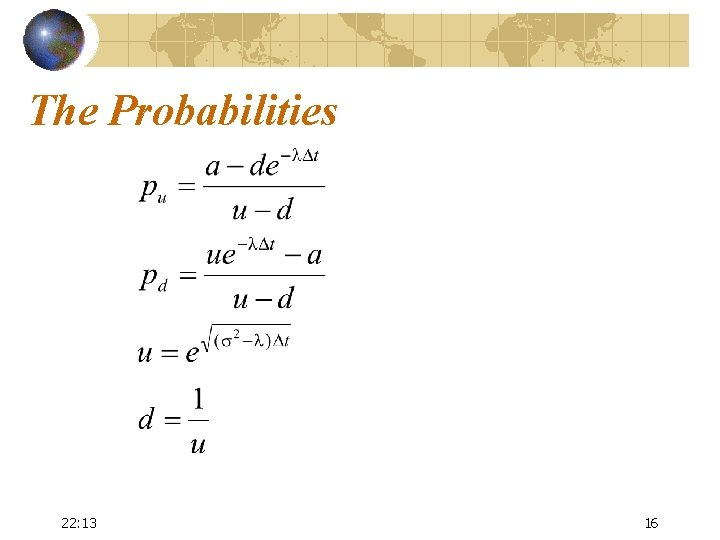The Probabilities 22: 13 16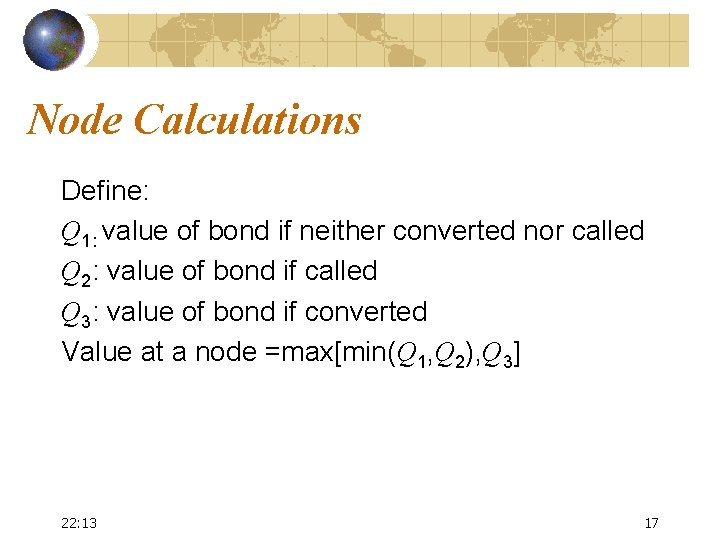Node Calculations Define: Q 1: value of bond if neither converted nor called Q 2: value of bond if called Q 3: value of bond if converted Value at a node =max[min(Q 1, Q 2), Q 3] 22: 13 17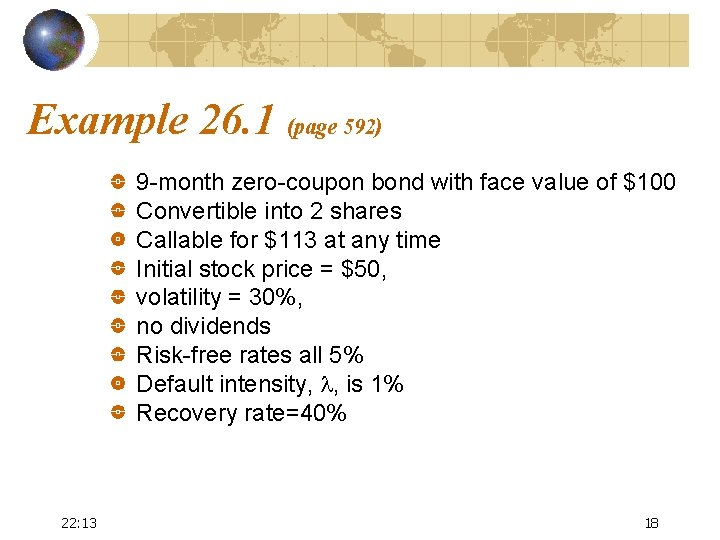Example 26. 1 (page 592) 9 -month zero-coupon bond with face value of \$100 Convertible into 2 shares Callable for \$113 at any time Initial stock price = \$50, volatility = 30%, no dividends Risk-free rates all 5% Default intensity, l, is 1% Recovery rate=40% 22: 13 18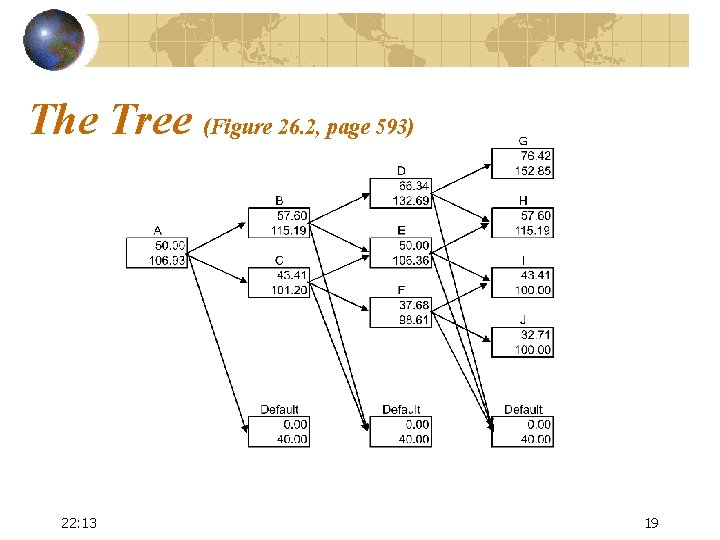The Tree (Figure 26. 2, page 593) 22: 13 19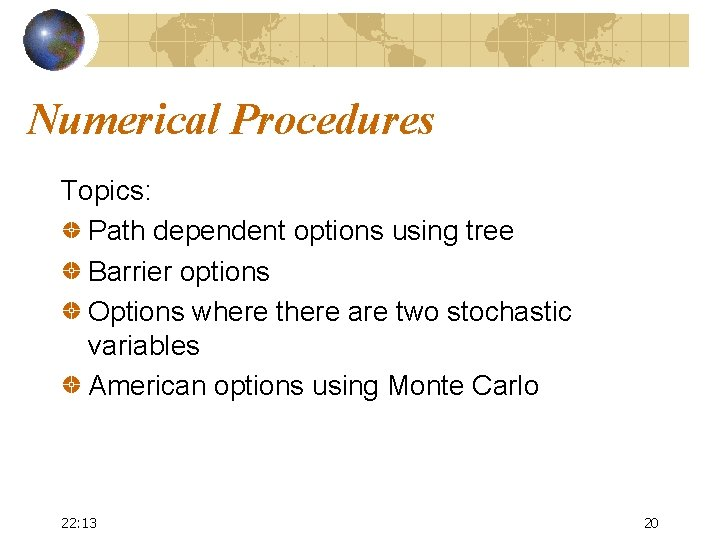Numerical Procedures Topics: Path dependent options using tree Barrier options Options where there are two stochastic variables American options using Monte Carlo 22: 13 20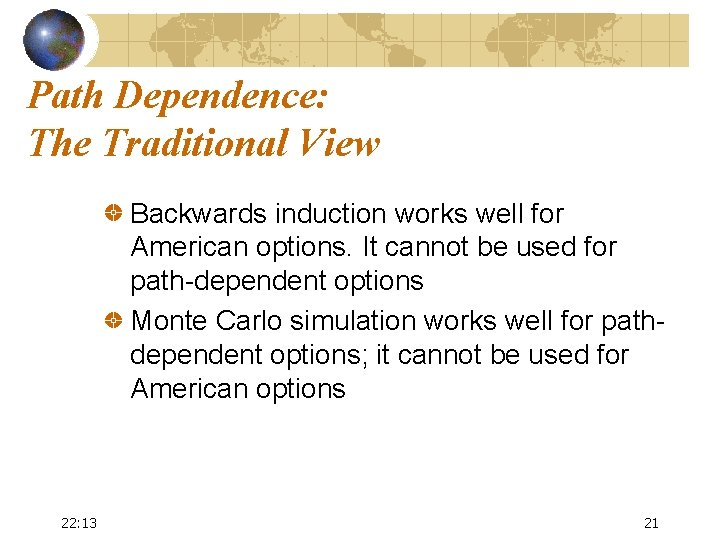Path Dependence: The Traditional View Backwards induction works well for American options. It cannot be used for path-dependent options Monte Carlo simulation works well for pathdependent options; it cannot be used for American options 22: 13 21Extension of Backwards Induction Backwards induction can be used for some path-dependent options We will first illustrate the methodology using lookback options and then show it can be used for Asian options 22: 13 22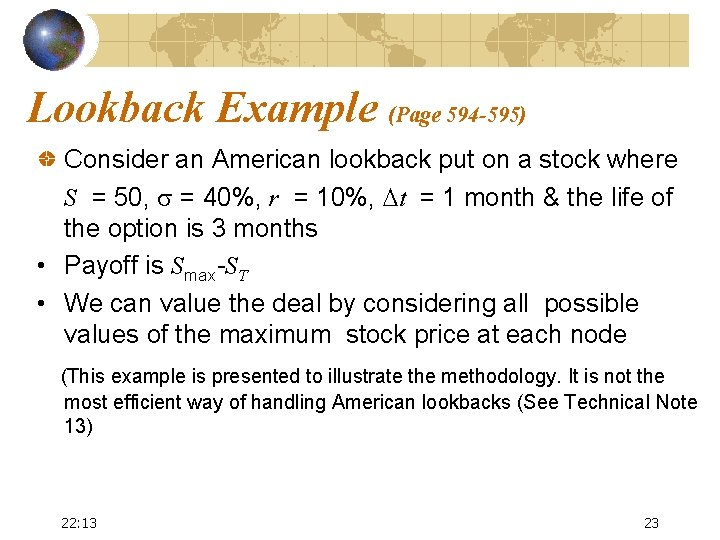Lookback Example (Page 594 -595) Consider an American lookback put on a stock where S = 50, s = 40%, r = 10%, Dt = 1 month & the life of the option is 3 months • Payoff is Smax-ST • We can value the deal by considering all possible values of the maximum stock price at each node (This example is presented to illustrate the methodology. It is not the most efficient way of handling American lookbacks (See Technical Note 13) 22: 13 23Example: An American Lookback Put Option (Figure 26. 3, page 595) S 0 = 50, s = 40%, r = 10%, Dt = 1 month, 70. 70 0. 00 62. 99 56. 12 50. 00 5. 47 56. 12 4. 68 44. 55 50. 00 62. 99 3. 36 56. 12 62. 99 56. 12 6. 87 50. 00 A 56. 12 50. 00 6. 12 2. 66 39. 69 44. 55 56. 12 50. 00 11. 57 5. 45 6. 38 50. 00 35. 36 10. 31 50. 00 14. 64 22: 13 24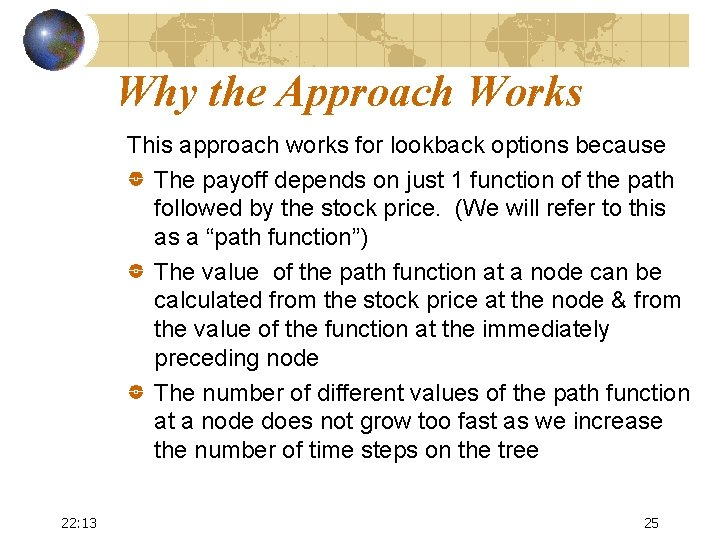Why the Approach Works This approach works for lookback options because The payoff depends on just 1 function of the path followed by the stock price. (We will refer to this as a “path function”) The value of the path function at a node can be calculated from the stock price at the node & from the value of the function at the immediately preceding node The number of different values of the path function at a node does not grow too fast as we increase the number of time steps on the tree 22: 13 25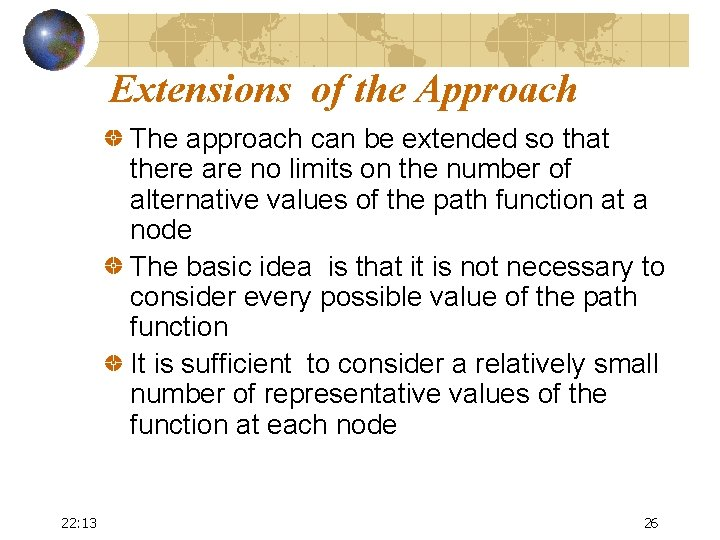Extensions of the Approach The approach can be extended so that there are no limits on the number of alternative values of the path function at a node The basic idea is that it is not necessary to consider every possible value of the path function It is sufficient to consider a relatively small number of representative values of the function at each node 22: 13 26Working Forward First work forward through the tree calculating the max and min values of the “path function” at each node Next choose representative values of the path function that span the range between the min and the max Simplest approach: choose the min, the max, and N equally spaced values between the min and max 22: 13 27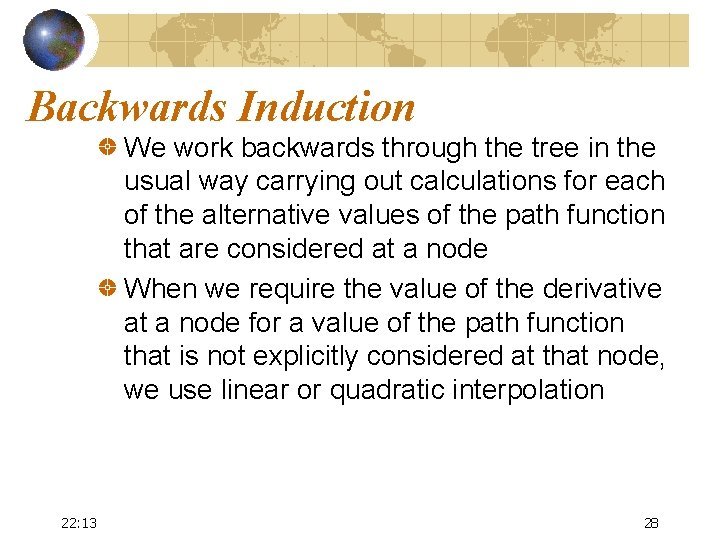Backwards Induction We work backwards through the tree in the usual way carrying out calculations for each of the alternative values of the path function that are considered at a node When we require the value of the derivative at a node for a value of the path function that is not explicitly considered at that node, we use linear or quadratic interpolation 22: 13 28Part of Tree to Calculate Value of an Option on the Arithmetic Average S = 54. 68 Y (Figure 26. 4, page 597) 0. 5056 Average S Option Price 47. 99 51. 12 54. 26 57. 39 7. 575 8. 101 8. 635 9. 178 S = 50. 00 Average S Option Price 46. 65 49. 04 51. 44 53. 83 5. 642 5. 923 6. 206 6. 492 X S = 45. 72 S=50, X=50, s=40%, r =10%, T=1 yr, Dt=0. 05 yr. We are at time 4 Dt 22: 13 Average S Option Price 0. 4944 Z 43. 88 46. 75 49. 61 52. 48 3. 430 3. 750 4. 079 4. 416 29Part of Tree to Calculate Value of an Option on the Arithmetic Average (continued) Consider Node X when the average of 5 observations is 51. 44 Node Y: If this is reached, the average becomes 51. 98. The option price is interpolated as 8. 247 Node Z: If this is reached, the average becomes 50. 49. The option price is interpolated as 4. 182 Node X: value is (0. 5056× 8. 247 + 0. 4944× 4. 182)e– 0. 1× 0. 05 = 6. 206 22: 13 30Using Trees with Barriers (Section 26. 6, page 598) When trees are used to value options with barriers, convergence tends to be slow The slow convergence arises from the fact that the barrier is inaccurately specified by the tree 22: 13 31True Barrier vs Tree Barrier for a Knockout Option: The Binomial Tree Case Tree Barrier True Barrier 22: 13 32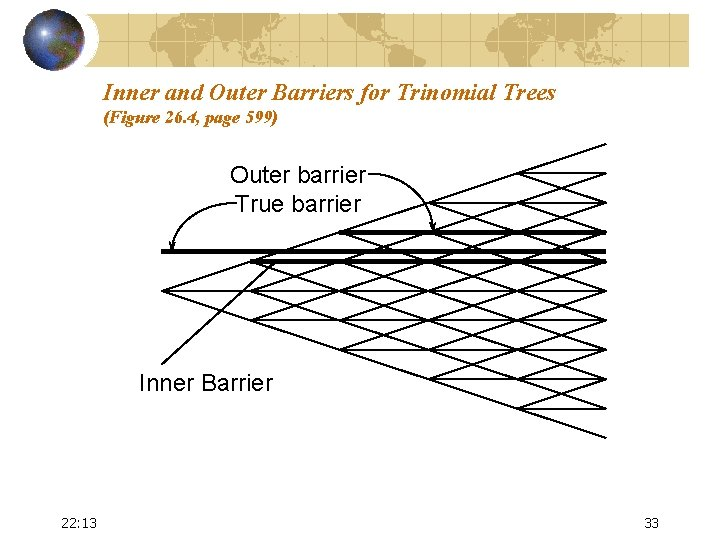Inner and Outer Barriers for Trinomial Trees (Figure 26. 4, page 599) Outer barrier True barrier Inner Barrier 22: 13 33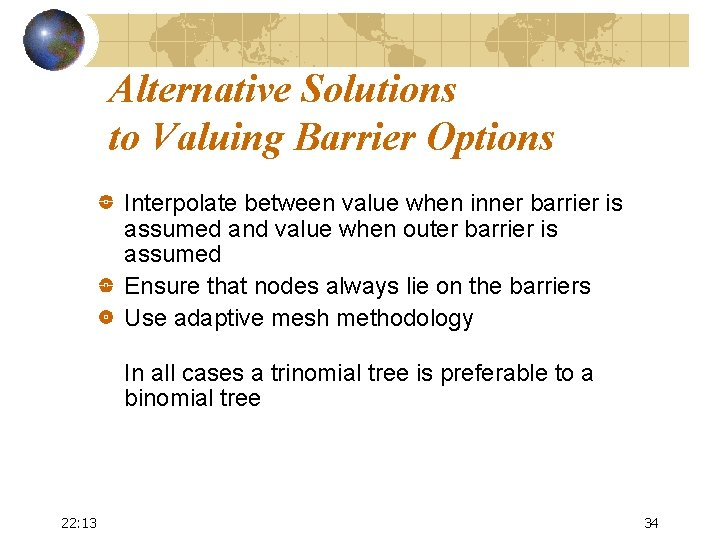Alternative Solutions to Valuing Barrier Options Interpolate between value when inner barrier is assumed and value when outer barrier is assumed Ensure that nodes always lie on the barriers Use adaptive mesh methodology In all cases a trinomial tree is preferable to a binomial tree 22: 13 34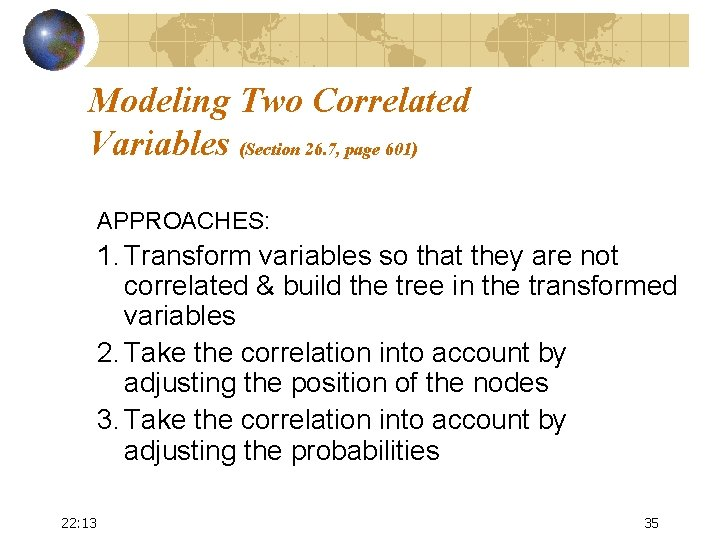Modeling Two Correlated Variables (Section 26. 7, page 601) APPROACHES: 1. Transform variables so that they are not correlated & build the tree in the transformed variables 2. Take the correlation into account by adjusting the position of the nodes 3. Take the correlation into account by adjusting the probabilities 22: 13 35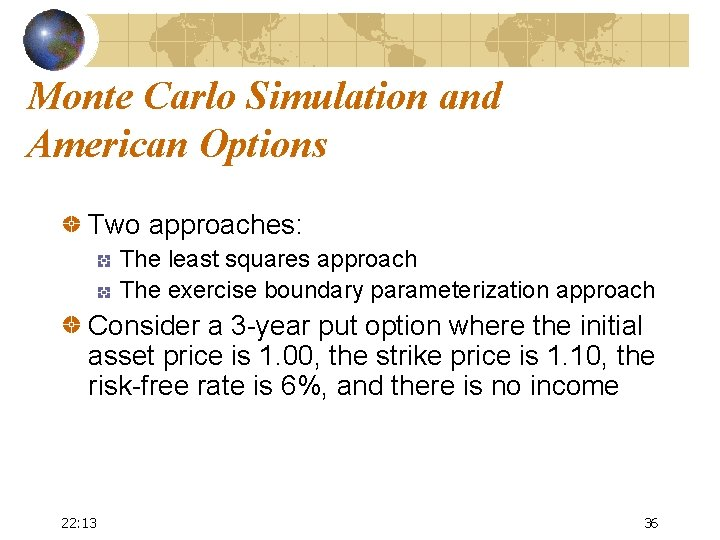Monte Carlo Simulation and American Options Two approaches: The least squares approach The exercise boundary parameterization approach Consider a 3 -year put option where the initial asset price is 1. 00, the strike price is 1. 10, the risk-free rate is 6%, and there is no income 22: 13 36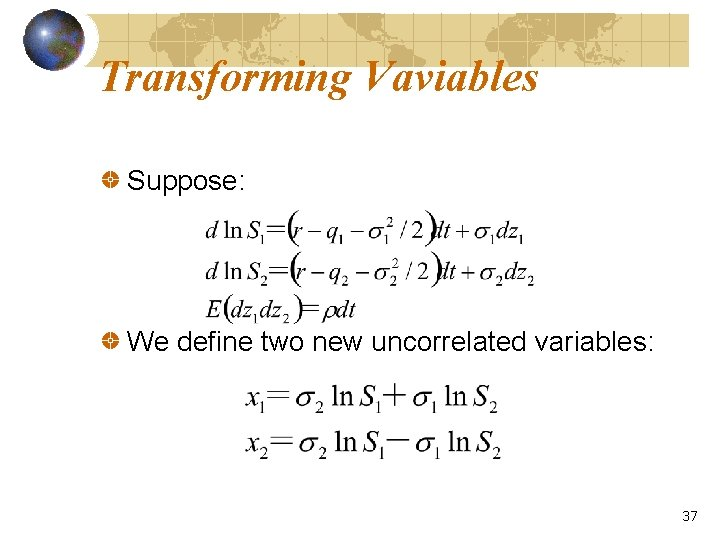Transforming Vaviables Suppose: We define two new uncorrelated variables: 37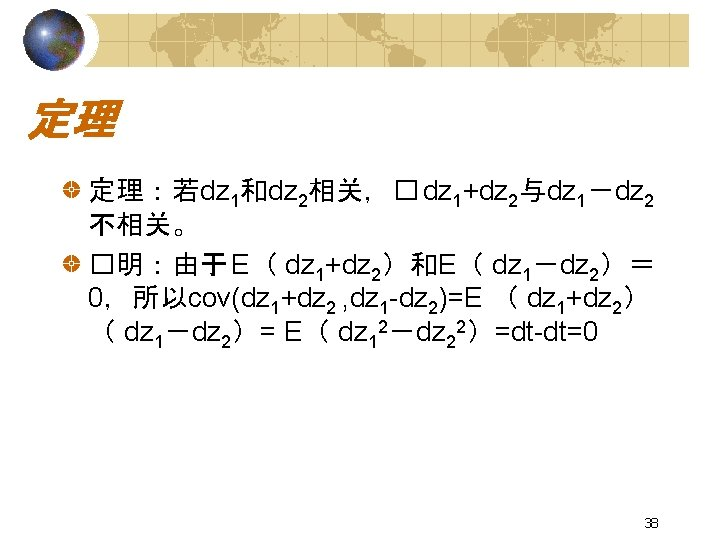From P 30 39Sampled Paths Path t = 0 22: 14 t =1 t =2 t =3 1 1. 00 1. 09 1. 08 1. 34 2 1. 00 1. 16 1. 26 1. 54 3 1. 00 1. 22 1. 07 1. 03 4 1. 00 0. 93 0. 97 0. 92 5 1. 00 1. 11 1. 56 1. 52 6 1. 00 0. 76 0. 77 0. 90 7 1. 00 0. 92 0. 84 1. 01 8 1. 00 0. 88 1. 22 1. 34 41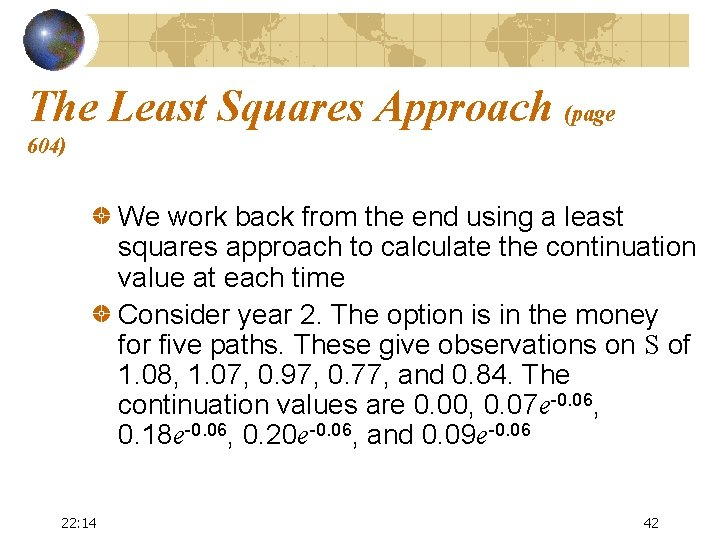The Least Squares Approach (page 604) We work back from the end using a least squares approach to calculate the continuation value at each time Consider year 2. The option is in the money for five paths. These give observations on S of 1. 08, 1. 07, 0. 97, 0. 77, and 0. 84. The continuation values are 0. 00, 0. 07 e-0. 06, 0. 18 e-0. 06, 0. 20 e-0. 06, and 0. 09 e-0. 06 22: 14 42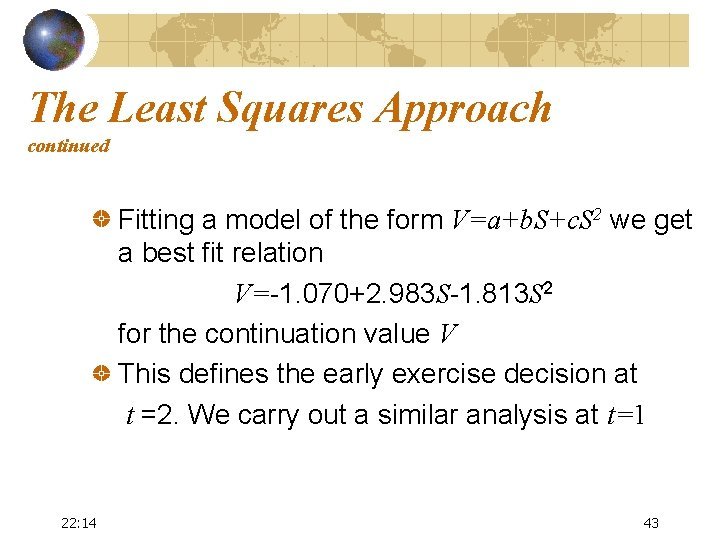The Least Squares Approach continued Fitting a model of the form V=a+b. S+c. S 2 we get a best fit relation V=-1. 070+2. 983 S-1. 813 S 2 for the continuation value V This defines the early exercise decision at t =2. We carry out a similar analysis at t=1 22: 14 43The Least Squares Approach continued In practice more complex functional forms can be used for the continuation value and many more paths are sampled 22: 14 44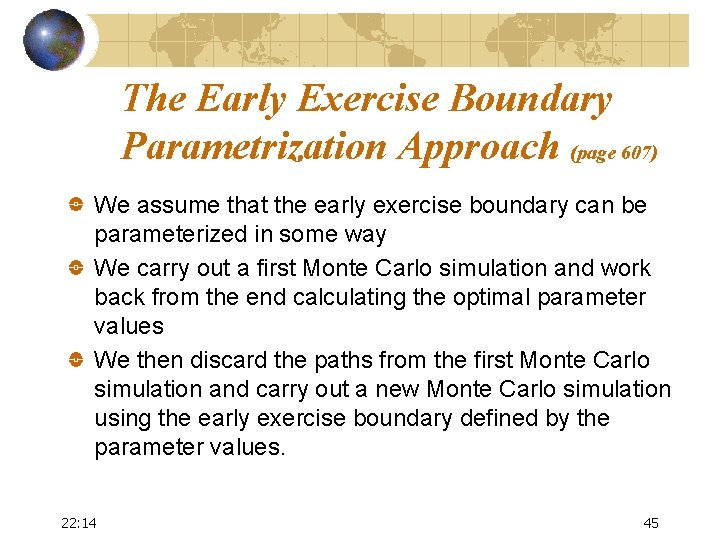The Early Exercise Boundary Parametrization Approach (page 607) We assume that the early exercise boundary can be parameterized in some way We carry out a first Monte Carlo simulation and work back from the end calculating the optimal parameter values We then discard the paths from the first Monte Carlo simulation and carry out a new Monte Carlo simulation using the early exercise boundary defined by the parameter values. 22: 14 45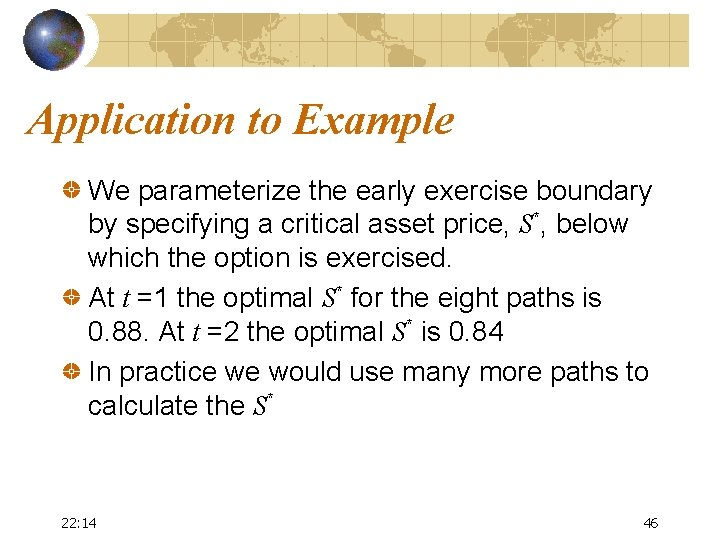Application to Example We parameterize the early exercise boundary by specifying a critical asset price, S*, below which the option is exercised. At t =1 the optimal S* for the eight paths is 0. 88. At t =2 the optimal S* is 0. 84 In practice we would use many more paths to calculate the S* 22: 14 46